﻿ Notes on Several Families of Differential Equations Related to the Generating Function for the Bernoulli Numbers of the Second KindPublications are Open
Access in this journal
Article Versions
Export Article
• Normal Style
• MLA Style
• APA Style
• Chicago Style
Original Article
Open Access Peer-reviewed

Notes on Several Families of Differential Equations Related to the Generating Function for the Bernoulli Numbers of the Second Kind

Feng Qi
Turkish Journal of Analysis and Number Theory. 2018, 6(2), 40-42. DOI: 10.12691/tjant-6-2-1
Received December 08, 2017; Revised February 02, 2018; Accepted March 15, 2018

Abstract

In the paper, the author notes several families of ordinary differential equations related to the generating function for the Bernoulli numbers of the second kind.

It is well known 1, 2, 3, 4 that the Bernoulli numbers of the second kind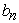for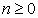can be generated by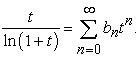This means that the reciprocal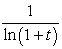of the logarithmic function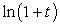is related to the Bernoulli numbers of the second kindfor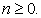In [ 5, Theorem 2.1], it was obtained inductively and recursively that the nonlinear differential equations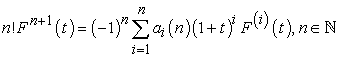(1)

have a solution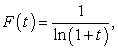where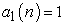and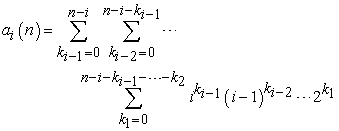(2)

for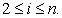In [ 6, Lemma 2.1], it was obtained also inductively and recursively that the family of differential equations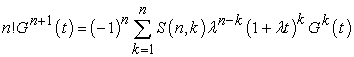(3)

for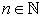has a solution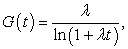where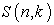denotes the Stirling numbers of the second kind. In [ 6, Corollary 2.2], it was claimed that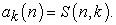(4)

In [ 7, Theorem 1], it was established still inductively and recursively that the family of differential equations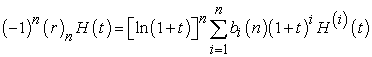forhas a solution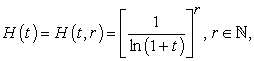where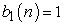and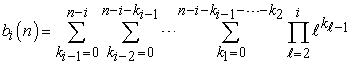(5)

for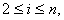and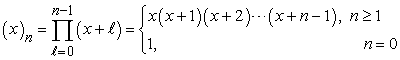is called 8 the rising factorial.

The proofs of [ 7, Theorem 1], [ 5, Theorem 2.1], and [ 6, Lemma 2.1] are almost the same and have the same shortcoming: they are rather long and tedious. More importantly, the expressions (2) and (5) are difficult to be understood, to be remembered, and to be computed.

By virtue of the Faà di Bruno formula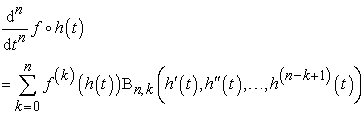for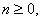where the Bell polynomials of the second kind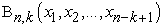for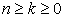are defined [ 9, p. 134, Theorem A] and [ 9, p. 139, Theorem C] by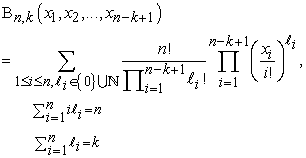in view of two identities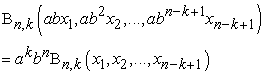and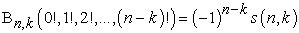in [ 9, p. 135], where a and b are any complex numbers and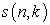stands for the Stirling numbers of the first kind, and in light of an inversion theorem [ 10, p. 171, Theorem 12.1] which reads that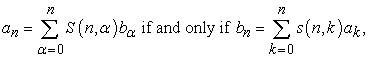(6)

two families of differential equations(7)

and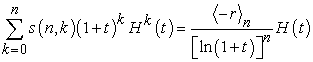(8)

forand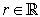were standardly established in [ 11, Theorem 1], where the falling factorial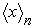of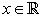for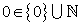is defined 8 by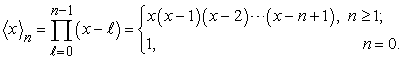Since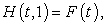taking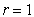in (8) derives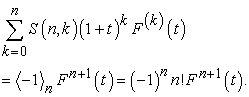(9)

Comparing (9) with (1) results in the equality (4). Replacing t by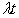in (9) yields the differential equation (3).

Letting r = 1 in (7) arrives at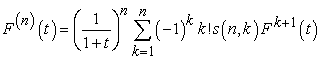(10)

for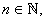which is an inversion formula of (1). This can also be deduced from applying (6) to (1).

Replacing t byin (10) gives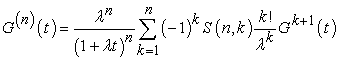forwhich is an inversion formula of (3). This can also be acquired by applying (6) to (3).

Remark. The motivations in the papers 4, 11, 12, 13, 14, 15, 16, 17, 18, 19, 20, 21, 22, 23, 24, 25, 26, 27, 28, 29, 30, 31, 32, 33, 34, 35, 36 are same as the one in this paper. This paper is a slightly modified version of the preprint 37.

Acknowledgements

The author thanks Professor D. V. Dolgy at Far Eastern Federal University and Kwangwoon University for his e-mailing the paper 4 to the author on 7 December 2017.

References

  F. Qi, A new formula for the Bernoulli numbers of the second kind in terms of the Stirling numbers of the first kind, Publ. Inst. Math. (Beograd) (N.S.) 100(114) (2016), 243-249. In article View Article  F. Qi, Explicit formulas for computing Bernoulli numbers of the second kind and Stirling numbers of the first kind, Filomat 28 (2014), no. 2, 319-327. In article View Article  F. Qi and X.-J. Zhang, An integral representation, some inequalities, and complete monotonicity of the Bernoulli numbers of the second kind, Bull. Korean Math. Soc. 52 (2015), no. 3, 987-998. In article View Article  F. Qi and J.-L. Zhao, Some properties of the Bernoulli numbers of the second kind and their generating function, Research Gate Working Paper (2017). In article View Article  T. Kim, D. S. Kim, H. I. Kwon, and J. J. Seo, Revisit nonlinear differential equations associated with Bernoulli numbers of the second kind, Glob. J. Pure Appl. Math. 12 (2016), 1893-1901. In article View Article  S.-S. Pyo, T. Kim, and S.-H. Rim, Identities of the degenerate Daehee numbers with the Bernoulli numbers of the second kind arising from nonlinear differential equation, J. Nonlinear Sci. Appl. 10 (2017), no. 12, 6219-6228. In article View Article  D. V. Dolgy, L.-C. Jang, D.-S. Kim, T.-K. Kim, and J.-J. Seo, Differential equations associated with higher-order Bernoulli numbers of the second kind revisited, J. Anal. Appl. 14 (2016), no. 2, 107-121. In article  F. Qi, X.-T. Shi, and F.-F. Liu, Several identities involving the falling and rising factorials and the Cauchy, Lah, and Stirling numbers, Acta Univ. Sapientiae Math. 8 (2016), no. 2, 282-297. In article View Article  L. Comtet, Advanced Combinatorics: The Art of Finite and Infinite Expansions, Revised and Enlarged Edition, D. Reidel Publishing Co., Dordrecht and Boston, 1974. In article View Article  J. Quaintance and H. W. Gould, Combinatorial Identities for Stirling Numbers. The unpublished notes of H. W. Gould. With a foreword by George E. Andrews. World Scientific Publishing Co. Pte. Ltd., Singapore, 2016. In article  F. Qi, D.-W. Niu, and B.-N. Guo, Simplifying coefficients in differential equations associated with higher order Bernoulli numbers of the second kind, Preprints 2017, 2017080026, 6 pages. In article View Article  F. Qi, A simple form for coefficients in a family of nonlinear ordinary differential equations, Research Gate Preprint (2017). In article View Article  F. Qi, A simple form for coefficients in a family of ordinary differential equations related to the generating function of the Legendre polynomials, Research Gate Preprint (2017). In article View Article  F. Qi, Explicit formulas for the convolved Fibonacci numbers, ResearchGate Working Paper (2016). In article View Article  F. Qi, Simple forms for coefficients in two families of ordinary differential equations, Glob. J. Math. Anal. 6 (2018), no. 1, 7-9. In article View Article  F. Qi, Simplification of coefficients in two families of nonlinear ordinary differential equations, Research Gate Preprint (2017). In article View Article  F. Qi, Simplifying coefficients in a family of nonlinear ordinary differential equations, Acta Comment. Univ. Tartu. Math. (2018), in press; ResearchGate Preprint (2017). In article View Article  F. Qi, Simplifying coefficients in a family of ordinary differential equations related to the generating function of the Laguerre polynomials, ResearchGate Preprint (2017). In article View Article  F. Qi, Simplifying coefficients in a family of ordinary differential equations related to the generating function of the Mittag-Leffer polynomials, Research Gate Preprint (2017). In article View Article  F. Qi, Simplifying coefficients in differential equations related to generating functions of reverse Bessel and partially degenerate Bell polynomials, Research Gate Preprint (2017). In article View Article  F. Qi, The inverse of a matrix and several identities related to the Catalan numbers and the Chebyshev polynomials of the second kind, Research Gate Presentation (2017). In article View Article  F. Qi and B.-N. Guo, A diagonal recurrence relation for the Stirling numbers of the first kind, Appl. Anal. Discrete Math. 12 (2018), no. 1, in press. In article View Article  F. Qi and B.-N. Guo, Explicit formulas and recurrence relations for higher order Eulerian polynomials, Indag. Math. 28 (2017), no. 4, 884-891. In article View Article  F. Qi and B.-N. Guo, Some properties of the Hermite polynomials and their squares and generating functions, Preprints 2016, 2016110145, 14 pages. In article View Article  F. Qi and B.-N. Guo, Viewing some ordinary differential equations from the angle of derivative polynomials, Preprints 2016, 2016100043, 12 pages. In article View Article  F. Qi, D. Lim, and B.-N. Guo, Explicit formulas and identities for the Bell polynomials and a sequence of polynomials applied to differential equations, Rev. R. Acad. Cienc. Exactas Fís. Nat. Ser. A Mat. RACSAM (2018), in press. In article View Article  F. Qi, D. Lim, and B.-N. Guo, Some identities relating to Eulerian polynomials and involving Stirling numbers, Preprints 2017, 2017080004, 10 pages. In article View Article  F. Qi, D. Lim, and A.-Q. Liu, Explicit expressions related to degenerate Cauchy numbers and their generating function, HAL archives (2018). In article View Article  F. Qi, D.-W. Niu, and B.-N. Guo, Simplification of coefficients in differential equations associated with higher order Frobenius-Euler numbers, Preprints 2017, 2017080017, 7 pages. In article View Article  F. Qi, D.-W. Niu, and B.-N. Guo, Some identities for a sequence of unnamed polynomials connected with the Bell polynomials, Rev. R. Acad. Cienc. Exactas Fís. Nat. Ser. A Math. RACSAM 112 (2018), in press. In article View Article  F. Qi, X.-L. Qin, and Y.-H. Yao, The generating function of the Catalan numbers and lower triangular integer matrices, Preprints 2017, 2017110120, 12 pages. In article View Article  F. Qi, J.-L. Wang, and B.-N. Guo, Notes on a family of inhomogeneous linear ordinary differential equations, Adv. Appl. Math. Sci. 17 (2018), no. 4, 361-368. In article View Article  F. Qi, J.-L. Wang, and B.-N. Guo, Simplifying and finding nonlinear ordinary differential equations, ResearchGate Working Paper (2017). In article View Article  F. Qi, J.-L. Wang, and B.-N. Guo, Simplifying differential equations concerning degenerate Bernoulli and Euler numbers, Trans. A. Razmadze Math. Inst. 172 (2018), no. 1, 90-94. In article View Article  F. Qi, Q. Zou, and B.-N. Guo, Some identities and a matrix inverse related to the Chebyshev polynomials of the second kind and the Catalan numbers, Preprints 2017, 2017030209, 25 pages. In article View Article  J.-L. Zhao, J.-L. Wang, and F. Qi, Derivative polynomials of a function related to the Apostol-Euler and Frobenius-Euler numbers, J. Nonlinear Sci. Appl. 10 (2017), no. 4, 1345-1349. In article View Article  F. Qi, Notes on several families of differential equations related to the generating function for the Bernoulli numbers of the second kind, ResearchGate Preprint (2017). In article View ArticleThis work is licensed under a Creative Commons Attribution 4.0 International License. To view a copy of this license, visit http://creativecommons.org/licenses/by/4.0/